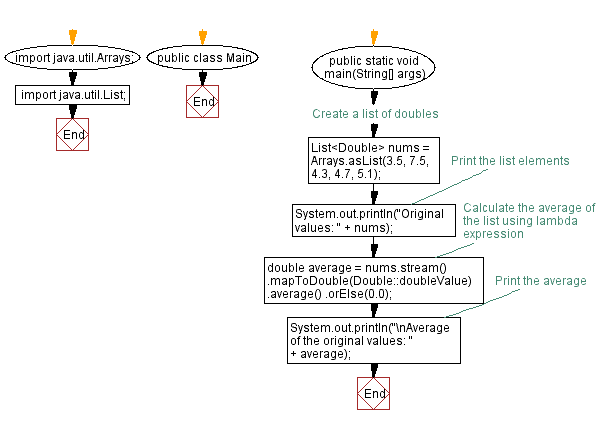# Calculate average of doubles using Lambda expression in Java

## Java Lambda Program: Exercise-6 with Solution

Write a Java program to implement a lambda expression to find the average of a list of doubles.

Sample Solution:

Java Code:

``````import java.util.Arrays;
import java.util.List;

public class Main {
public static void main(String[] args) {
// Create a list of doubles
List<Double> nums = Arrays.asList(3.5, 7.5, 4.3, 4.7, 5.1);
// Print the list elements
System.out.println("Original values: " + nums);

// Calculate the average of the list using lambda expression
double average = nums.stream()
.mapToDouble(Double::doubleValue)
.average()
.orElse(0.0);

// Print the average
System.out.println("\nAverage of the original values: " + average);
}
}
``````

Sample Output:

```Original values: [3.5, 7.5, 4.3, 4.7, 5.1]

Average of the original values: 5.0200000000000005
```

Explanation:

To calculate the average of the list, we use the stream() method on the nums list to convert it into a stream. Then, we use the mapToDouble() method to convert each Double object to its corresponding primitive double value. This step is necessary because the average() method operates on primitive double values.

Next, we call the average() method to calculate the average of the stream. If the stream is empty, we use orElse(0.0) to provide a default value of 0.0.

Flowchart:Live Demo:

Java Code Editor:

Improve this sample solution and post your code through Disqus

Java Lambda Exercises Previous: Sort strings in alphabetical order using Lambda expression in Java.
Java Lambda Exercises Next: Remove duplicates from list of integers using Lambda expression in Java.

What is the difficulty level of this exercise?

Test your Programming skills with w3resource's quiz.

﻿

## Java: Tips of the Day

distinctValuesOfArray

Returns all the distinct values of an array.

Uses Arrays.stream().distinct() to discard all duplicated values.

```public static int[] distinctValuesOfArray(int[] elements) {
return Arrays.stream(elements).distinct().toArray();
}
```

Ref: https://bit.ly/3mn5ner

We are closing our Disqus commenting system for some maintenanace issues. You may write to us at reach[at]yahoo[dot]com or visit us at Facebook